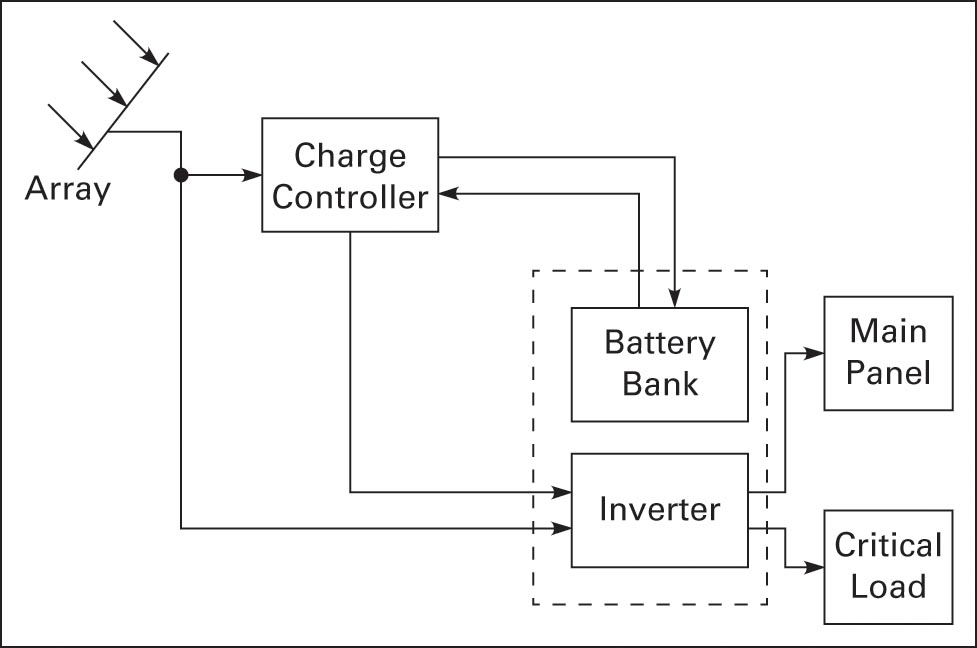# Battery Bank Sizing

Want create site? Find Free WordPress Themes and plugins.

The following steps can be used to estimate the size of a battery bank for a stand-alone PV system (and other systems).

1. Calculate the average daily AC load at Wh per day (this is done before the battery bank sizing by using utility electric bills or adding the watts for each item using power).

2. Divide step 1 by inverter efficiency.

3. Divide step 2 by system DC voltage.

4. Step 3 is the average (required) Ah per day.

5. Multiply step 4 by days of autonomy.

6. If using lead-acid batteries, multiply step 5 by the temperature multiplier (see note 1).

7. Divide step 6 by percent DOD (if not using lead-acid batteries, divide step 5 by percent DOD).

8. Divide step 7 by (selected for the system) battery Ah rating.

9. Step 8 is the number of batteries required in parallel.

10. Divide system DC voltage by battery voltage (see note 2).

11. Step 10 is the number of batteries required in a series string.

12. Multiply step 11 by step 8.

13. Step 12 is the total number of batteries required in the battery bank.

Note 1: This is a derating factor based on the lowest ambient temperature of the battery bank and is usually in the battery specification.

Note 2: As an example, the system DC voltage is selected as 24V and the battery voltage as 12V; therefore, two batteries per series string are required. This criterion is part of the design selection process.

## Battery Bank Sizing Example

Design a battery bank backup system for a PV- array stand-alone system (see Figure 1). The system output is AC supplied by an inverter. Batteries will supply power on days of autonomy and at night.

• Load requirement: 14 kWh per day

• Days of autonomy: 2

• Battery system: 24V

• Battery spec.:12V at 250 Ah lead-acid deep-cycle

• DOD: 70%

• Lowest ambient temperature: 40°F (1.3 temperature multiplier)

• Inverter efficiency: 90%

Note: In Figure 1, the area for battery bank sizing is within the dotted line. The array must supply power to meet the charging and system requirements when the array is generating power, and this is calculated when the array size is determined. When sufficient power cannot be generated by the array to meet total demand, then only critical loads will be supplied. In this circumstance, a motor generator is often used to supplement power requirements.Figure 1 Stand-alone configuration with battery backup

Did you find apk for android? You can find new Free Android Games and apps.